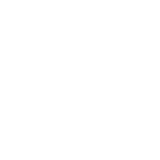Categories

# ISC 2020 Computer Science Theory Circular Queue Program

A Circular Queue is a linear data structure which works on the principle of FIFO, enables the user to enter data from the rear end and remove data from the front end with the rear end connected to the front end to form a circular pattern.

Define a class CirQueue with the following details:

Class name: CirQueue
Data members/instance variables:
cq[]: array to store integers.
cap: stores the maximum capacity of the array.
front: to point the index of the front end.
rear: to point the index of the rear end.
Member functions:
CirQueue(int max): constructor to initialize the data member cap = max, front = 0 and rear = 0.
void push(int n): to add integer in the queue from the rear end if possible, otherwise display the message “QUEUE IS FULL”.
int pop(): removes and returns the integer from the front end of the queue if any, else returns -9999.
void show(): displays the queue elements.

(a) Specify the class CirQueue giving details of the functions void push(int) and int pop(). Assume that the other functions have been defined.

The main function and algorithm need not be written.

(b) How is a linear queue structure different from a circular queue structure?

``````import java.io.*;
class CirQueue{
int cq[];
int cap;
int front;
int rear;
public CirQueue(int max){
cap = max;
front = 0;
rear = 0;
cq = new int[cap];
}
public void push(int n){
if((rear + 1) % cap != front){
rear = (rear + 1) % cap;
cq[rear] = n;
}
else
System.out.println("QUEUE IS FULL");
}
public int pop(){
if(front != rear){
int n = cq[front];
front = (front + 1) % cap;
return n;
}
else
return -9999;
}
public void show(){
if(front != rear){
for(int i = front; i <= rear; i = (i + 1) % cap)
System.out.print(cq[i] + "\t");
System.out.println();
}
else
System.out.println("QUEUE IS EMPTY");
}
}``````

Linear Queue Structure is different from a Circular Queue Structure because in a Circular Queue Structure, the front and the rear ends are connected.